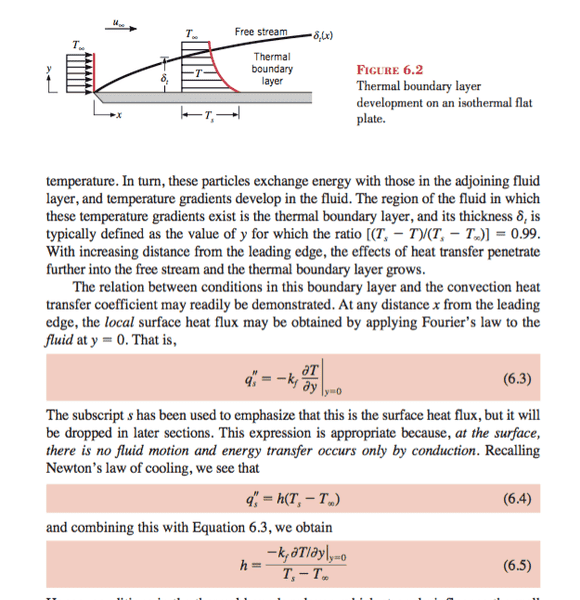# Convective heat transfer

Hello,
Consider this exemple:At y = 0 It is said that the heat is transferred by conduction because the fluid has a velocity equal to 0 (no-slip conditions). But the temperature of the fluid for y=0 is equal to Ts(temperature of the surface) so how come there's a gradient in the temperature? Do they mean there's gradient at y=0 just above the layer of the fluid having a temperature equal to Ts? Another question, the conduction heat transfer at y = 0 is happening for 1s (J/S = W) but during this second the fluid is passing and it is not static like in a wall that issue is confusing me. Even the exchange of energy between the fluid that is not on the surface, this fluid is flowing and heat is transferred each second by qs = h(Ts-Tinf) and on each position x we have a gradient of temperature that is independent of the time. I am very confused about the steady state heat transfer by convection.

Hope someone can clarify it

Thank you

Geofleur
Gold Member
Yes, the thermal gradient they are referring to is for ## y \geq 0 ##. Think of the fluid as an infinite heat sink of temperature ## T_\infty ## and the wall as an infinite heat reservoir of temperature ## T_s ##. Heat will flow from the reservoir to the sink. Right at the wall, heat is delivered purely conductively. Just above ## y = 0 ##, however, there are two competing processes: flowing fluid is removing heat, while fluid just below is delivering heat from the wall. At steady state, these two processes just balance one another, so that the temperature at any given point stays constant in time.

Note that steady state does not imply that the fluid is static. In fact, for there to be a steady state in this situation, the fluid must be moving. Otherwise, there would just be a hot conductive front penetrating further and further into the fluid as time progresses.

Great. but for exemple, heat flux is joule per second, but during this second the fluid will flow but how the temperature will remain constant for a position x

Geofleur
•